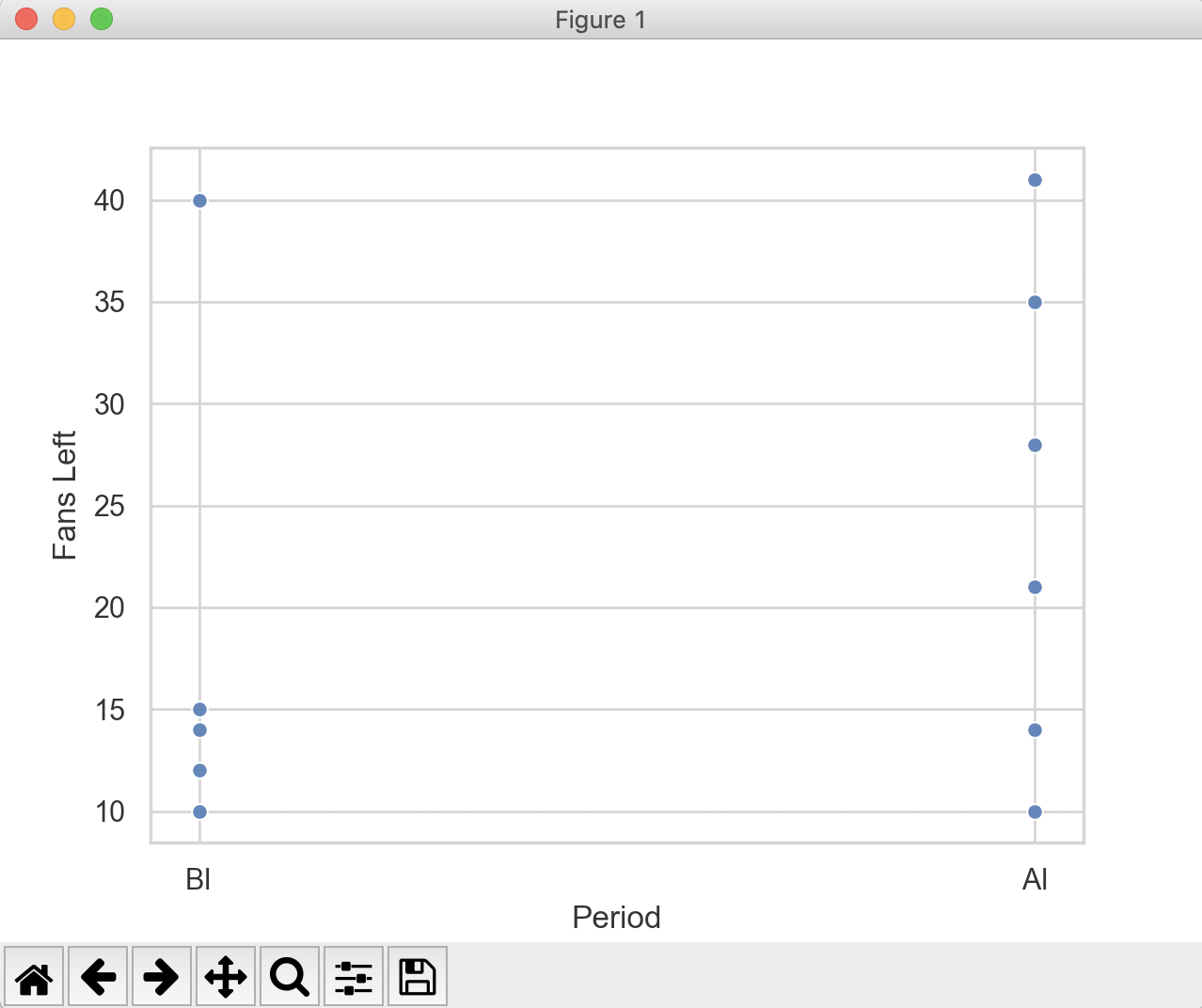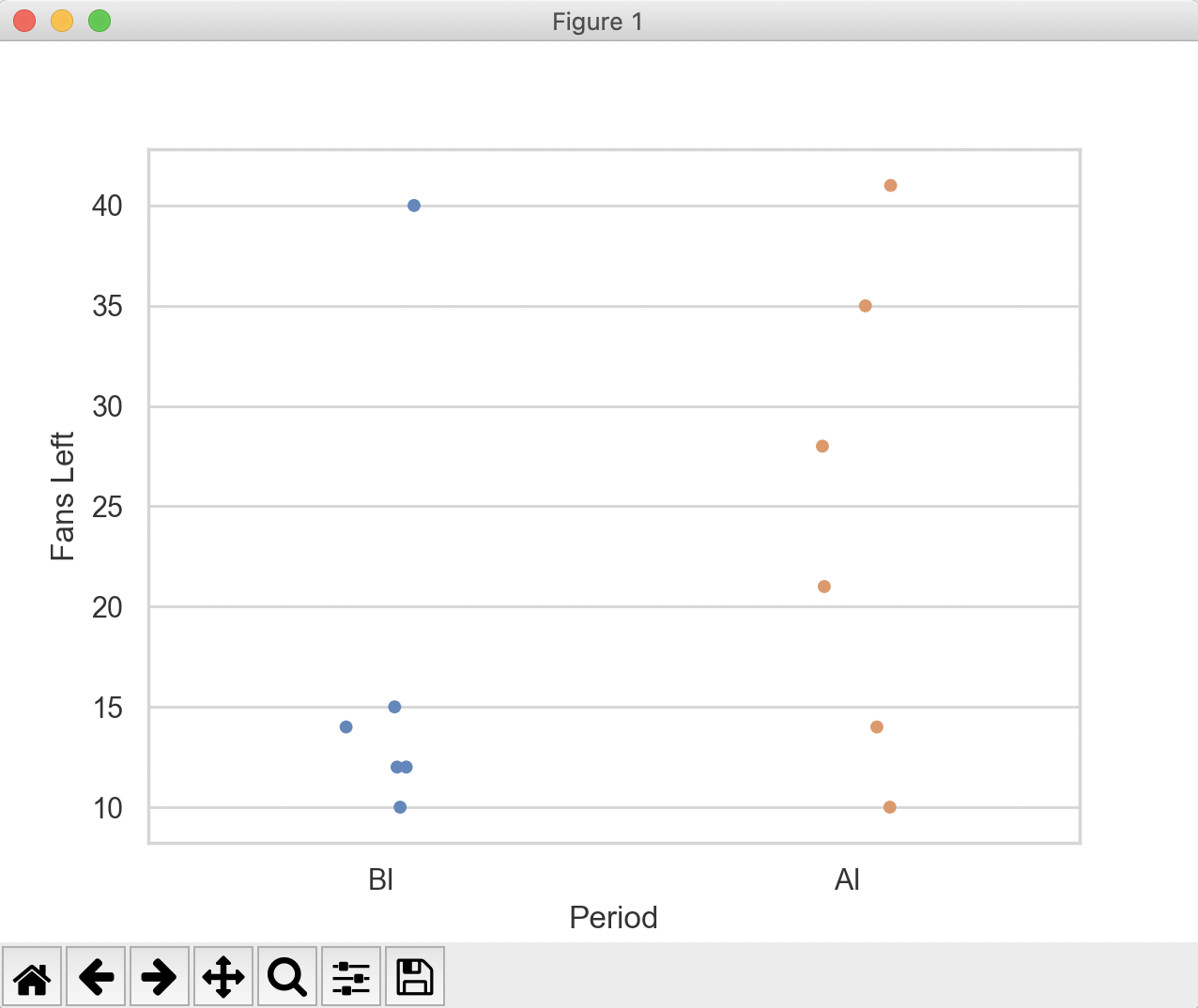# Drawing A Strip Plot Using Seaborn

## Overview:

• A scatter plot is employed to find out if there is any relationship between two variables.
• In a scatter plot, when both the variables are continous the markers find their places all over the plot.
• On the other hand, if one of the axis of a scatter plot represents a categorical variable(Not a continous variable - a discrete variable, a countable variable), the markers of a specific category align in a straigt line parallel to the other axis.
• To explicitly convey that the markers for each category are just clouds of values and not really a relation like straight line, a jitter plot is used.
• A jitter plot in this context, is a scatter plot drawn with a jitter value. The jitter value is between 0 to width of the category/2.

## Example -  Scatter plot:

 import matplotlib.pyplot as plt import pandas as pds import seaborn as sbn sbn.set(style="whitegrid"); periodVsFansLeft = {"Fans Left":[10, 15, 12, 12, 40, 14, 35, 28, 41, 21, 14, 10],                        "Period":["BI", "BI", "BI", "BI", "BI", "BI", "AI", "AI", "AI", "AI", "AI", "AI"]}; df = pds.DataFrame(data=periodVsFansLeft); print(df); sbn.scatterplot(x="Period", y="Fans Left", data=df); plt.show();

## Output:## Example: Drawing a strip plot using seaborn:

Making the following code change to the above example - i.e., replacing the scatterplot() function call with a call to stripplot(), draws a stripplot.

 sbn.stripplot(x="Period", y="Fans Left", data=df);

## Output: